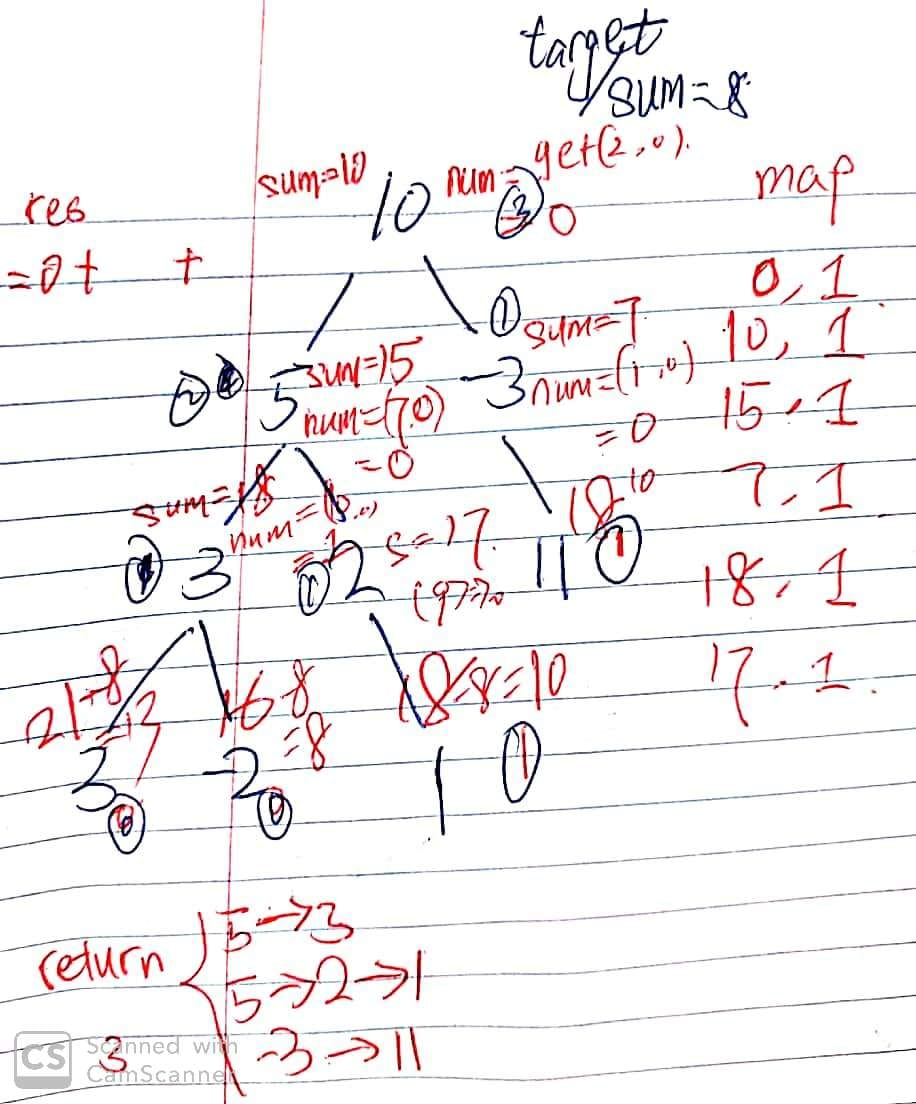# 437. Path Sum III

Reference: LeetCode
Difficulty: Easy

## Problem

You are given a binary tree in which each node contains an integer value. Find the number of paths that sum to a given value.

The path does not need to start or end at the root or a leaf, but it must go downwards (traveling only from parent nodes to child nodes).

The tree has no more than 1,000 nodes and the values are in the range -1,000,000 to 1,000,000.

Example:

• Reduce the time complexity.

## Analysis

By fudonglai:

pathSum 函数：给他一个节点和一个目标值，他返回以这个节点为根的树中，和为目标值的路径总数。
count 函数：给他一个节点和一个目标值，他返回以这个节点为根的树中，能凑出几个以该节点为路径开头，和为目标值的路径总数。

Methods:

1. Typical Recursion
• First, we should define each recursive function’s job.
• pathSum(x, target): Return the number of paths that satisfy target requirement in a tree whose root is x.
• count(x, target): Return the number of paths that satisfy target requirement in a tree whose root must be x, the head of those paths.
• Time: 11 ms
• In each level in tree, as you go down, $N$ is halved, and finally you call count(x, target) for the leaves whose cost is $T(1)$ (not all the same $N$). Each layer does $O(N)$, and in the best case there are $\log{N}$ layers.
• According to the master theorem,
• pathSum(): $T(N) = 2T(N/2) + O(N) = O(N\log{N})$
• count(): $T(N) = 2T(N/2) + O(1)$
• Best: $O(N\log{N})$
• Worst: $O(N^2)$
• Space: $O(h)$
2. HashMap + Prefix Sum (tankztc & kekezi)
• Time: $O(N)$ 4 ms
• Space: $O(N)$
• The idea is similar as Two Sum, using HashMap to store keys (the prefix sum) and values (how many ways to get to this prefix sum).
• The prefix sum stores the sum from the root to the current node in the recursion.
• Whenever we reach a node, we check if prefix sum - target exists in the HashMap.
• If it does, we added up the ways of prefix sum - target into result.
• For example:
• In one path we have: [1, 2, -1, -1, 2],
• then the prefix sum will be: [1, 3, 2, 1, 3].
• Let’s say we want to find target sum $2$, then we have $4$ paths which are 2, 1,2,-1, 2,-1,-1,2, and 2.
• The sum from any node in the middle of the path to the current node
• $=$ (the sum from the root to the current node) $-$ (the prefix sum of the node in the middle)
• We want the difference above equal to the target value. In addition, we need to know how many differences are equal to the target value.
• Use HashMap. The value of map stores the frequency of all possible sum in the path to the current node. If the difference we want exists, there must exist a node in the middle of the path, such that from this node to the current node, the sum is equal to the target value.
• There might be multiple nodes in the middle that satisfy the requirement. In each recursion, the map stores all information we need to calculate the number of ranges that sum up to target (start from a middle node and end by the current node).
• To get the total number of a path count, we add up the number of valid paths ended by each node in the tree.
• Each recursion returns the total count of valid paths in the subtree rooted at the current node. And this sum can be divided into three parts:
• The total number of valid paths in the subtree rooted at the current node’s left child.
• The total number of valid paths in the subtree rooted at the current node’s right child.
• The number of valid paths ended by the current node.
• The interesting part of this solution is that the prefix is counted from root to leaves, and the result of total count is calculated from the bottom to the top.

## Code

### Typical Recursion

#### Incorrect Version

First, let’s examine an incorrect version:

Example:

Call Stack:

We can see that there are repeated calculations.

• p(3, 3, 3) called by p(2, 2, 3) called by p(1, 3, 3).
• p(3, 3, 3) called by p(2, 3, 3) called by p(1, 3, 3).

### HashMap + Prefix Sum

Note:CommentJunhao Wang
a software engineering cat